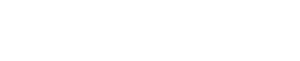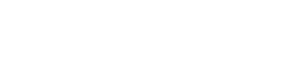# Mastering Arithmetic Sequences | Cambridge IGCSE Mathematics[Please watch the video attached at the end of this blog for a visual explanation of Mastering Arithmetic Sequences]

### Linear Sequences

A general linear sequence has the general form of un = an + b, where a and b are constants and un is the nth term.Let’s look at a more detailed example of what this is.

Ex: If we have the sequence 6, 10, 14, 18 and we are required to obtain the nth term, what is the process that we must follow?

Step 1: We put down the terms in a table. We know that these numbers given above are the first four terms of the sequence, so we write n = 1, 2, 3, 4.Step 2: Next to that we have to write each of the terms given as 6, 10, 14 and 18.Step 3: The next thing we have to see is the difference between each of the terms. In this example, we can see that you add 4 to the previous number to get the new number (6 + 4 = 10, 10 + 4 = 14, etc). Therefore, the value of a is +4.Step 3: Now that we have worked out the value of a, we have to work out the value of b. In order to do that, we can introduce another column to your table which is 4n. This means we are multiplying the n value here by 4. Therefore, the 4n values for n = 1, 2, 3, 4, will be 4, 8, 12, and 16 respectively.Step 4: Then we need to compare both the columns 4n and the value of a to see how much more we need to add to the 4n value for a to be the answer. In this example, it can be seen that we need to add 2 every time.So hence the final equation is un = 4n – 2.

Whereas in linear sequences, the first difference is always the same, in quadratic sequences, it is the second difference which is always the same.In quadratic sequences, what must be known is that the second difference is going to be equal to 2a.

We can consider an example sequence: 2, 7,14, 23, 34.

Step 1: Just like last time, we can put these values in a table and calculate the first difference between the numbers. In this case, we can see that the difference between the numbers is +5, +7, +9 and +11.Step 2: Once the first difference is calculated, the second difference also needs to be calculated. The second difference is the difference between consecutive numbers in the first difference (read that carefully, it might be a bit confusing!)

In this example we can see that the 1st difference between the numbers is +5, +7, +9 and +11. This means that the difference between two consecutive numbers here is +2.Step 3: Remember we said that 2a = the second difference. Therefore 2a = +2 (we found this out in the earlier step), and from here, we can work out the value of a = 1.

Step 4: Using the value of 1, we can substitute it into the common expression as un = n2 + bn + c, but we still need to work out the value of b and c. Therefore, we try to form two equations with 2 unknowns using the value of a.

We substitute the value of a as 1, and we get two equations

Equation 1; un = n2 + bn + c where n = 1,

2 = 1 + b + c

b + c = 1

Equation 2; un = n2 + bn + c where n = 2,

7 = 22 + 2b + c

7 = 4 + 2b + c

2b + c = 3Step 5: Use simultaneous equations to solve these two equations. In this case since both of these expressions have + c, we can just subtract both equations.

Equation 2 – Equation 1;

2b + c – ( b + c) = 3 – 1

b = 2

Substituting b in Equation 1;

b + c = 1

c = 1 – 2

c = -1

Step 6: Finally, substituting all the values we have found for a, b, and c, we can give the nth term in the equation form:

un = n2 + 2n – 1

### Cubic Sequences

Cubic sequences have a constant third difference.The third difference is equal to 6a.

Step 1: We repeat what we did in the previous sequences. We write down the n value, then the term value, and then we calculate not only the first difference, the second difference but the 3rd difference as well. We can see here that the 3rd difference is 6. Since the 3rd difference = 6a, the value of a = 1.Step 2: Once again, we can substitute the n values for 1, 2, and 3 this time because we need to have 3 variables and 3 unknowns to find out three particular equations. We can use simultaneous equations for this as well, but it is a bit different from what we did before.

Equation 1; where n = 1

un = an3 + bn2 + cn + d

4 = 1 + b + c + d

Equation 2; where n = 2,

16 = a23  + b22 + 2c + d

16 = 8a + 4b + 2c + d

16 = 8 + 4b + 2c + d

Equation 3; where n = 3

44 = a33 + b32 + 3c + d

44 = 27 + 9b + 3c + d

Step 3: The reason we use simultaneous equations is so we can remove one of the terms. Since d is common for all the equations, it is easier to use and remove d. So what we do is we subtract Equation 2 and Equation 1, and then we subtract Equations 3 and 2, so that when they are subtracted, we can identify a smaller equation which does not involve d.

Equation 2 – Equation 1;

16 – 4 = 8 – 1 + 4bb + 2c c + dd

12 = 7 + 3b + c

This is Equation 4

Equation 3 – Equation 2;

44 – 16 = 27 – 8 + 9b – 4b + 3c – 2c + dd

28 = 19 + 5b + c

This is Equation 5

Now we have two simultaneous equations as Equation 4 and Equation 5:

Equation 5 – Equation 4;

28 – 12 = 19 – 7 + 5b – 3b + c c

16 = 12 + 2b

2b = 4

b = 2

Substituting b in Equation 4;

12 = 7 + 3b + c

12 = 7 + 6 + c

c = -1.

Step 4: Now that the values for a, b, and c have been found, we can substitute these values in an equation to find the value of d.

Equation 1; 4 = 1 + b + c + d

4 = 1 + 2 + (-1) + d

4 = 2 + d

d = 2

Step 5: Once all the values of the constants have been worked out, the final equation for the nth term must be found out by substituting in these values.

un = n3 + 2n2 – 1n + 2

### Geometric Sequences

So the next thing is geometric sequences. Unlike arithmetic sequences where we consider the differences, geometric sequences are basically multiplication.  In such sequences, each term is obtained by multiplying the previous term by a constant r. The number r is known as the common ratio.Here we look at what we have to multiply from one term to get to the next term. In this case it is 3, therefore the nth term is 2 ✖ 3n-1.Mastering Arithmetic Sequences

Arithmetic sequences are really easy once you get the hang of it. Always make sure to study and memorise the general sequence and that you have subtracted, added or multiplied correctly.

If you are struggling with IGCSE revision or the Mathematics subject in particular, you can reach out to us at Tutopiya to join revision sessions or find yourself the right tutor for you.

Watch the video below for a visual explanation of the lesson on mastering arithmetic sequences. Don’t forget that there is a timed quiz to test whether you have understood the lesson!